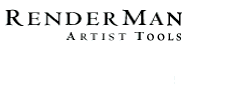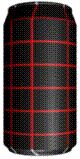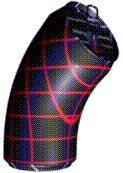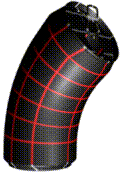PhotoRealistic RenderMan
Application Note #22

March, 1997

## Primitive Variables

It is often useful to attach data to the surface of an object. In RenderMan this is done by including the data as a token value pair when a surface primitive is declared. Once data is attached it is available as parameters to the shaders that are bound to the surface primitive. Parameters that are attached to surface geometry in this way are called primitive variables. Any parameter to a shader can be attached to surface geometry. For example, in the case of a bilinear patch:

```	Declare "bar" "constant float"
.
.
.
Surface "foo"
Patch "bilinear" "P" [-0.5 0.5 0  0.5 0.5 0
-0.5 -.5 0  0.5 -.5 0] "bar" [.5]
```

The primitive variable bar is now set to .5 and is constant over the entire patch. The shader foo is defined as follows:

```	surface foo (... float bar = 1.0;...)
{
.
.
.
}
```

The key word constant used in the Declare statement is called a class specifier. The next section introduces class specifiers.

## Class Specifiers

Class specifiers identify how a primitive variable will be interpolated over the uv parameter space of a surface primitive. The set of class specifiers for Photorealistic RenderMan is:

Constant: One value remains constant over the entire surface primitive.
Uniform: One value remains constant for each uv patch segment of the surface primitive.
Varying: Four values are interpolated over each uv patch segment of the surface. Bilinear interpolation is used for interpolation between the four values.
Vertex: Values are interpolated between each vertex in the surface primitive. The basis function of the surface is used for interpolation between vertices.
Facevarying: For polygons and subdivision surfaces, four values are interpolated over each face of the mesh. Bilinear interpolation is used for interpolation between the four values.

A variable's class and the surface primitive determine how many samples must be included when a primitive variable is attached to a surface primitive. The following two tables identify the correct number of values that must be included for each combination of class specifier and surface primitive.

Surface Primitive Constant Uniform Varying Vertex Facevarying
Quadrics 1 1 4 4 4
Polygon 1 1 nverts nverts nverts
GeneralPolygon 1 1 nverts nverts nverts
PointsPolygons 1 npolys nverts nverts sum(nverticesi)
PointsGeneralPolygons 1npolysnvertsnverts sum(nverticesi)
Points 1 1 npoints npoints npoints
SubdivisionMesh 1nfacesnvertsnverts sum(nverticesi)

Parametric Surface Primitives

Surface Primitive Constant Uniform Varying and Facevarying Vertex
Patch bilinear 1 1 4 4
Patch bicubic 1 1 4 16
PatchMesh bilinear 1 (nu-unowrap)*
(nv-vnowrap)
nu*nv nu*nv
PatchMesh bicubic 1usegs*vsegs(usegs+unowrap)*
(vsegs+vnowrap)
nu*nv
NuPatch 1(nu-uorder+1)*
(nv-vorder+1)
(nu-uorder+2)*
(nv-vorder+2)
nu*nv
Curves linear 1 sum(nsegsi-nowrap) sum(nverticesi) sum(nverticesi)
Curves cubic 1 sum(nsegsi) sum(nsegsi+nowrap) sum(nverticesi)
Blobby 11nleafnleaf

Note, in the above table for parametric surface primitives, unowrap, vnowrap, and nowrap are assumed to be a boolean value of either 0 = periodic or 1 = nonperiodic. This is NOT how uwrap, vwrap, and wrap are used in the RenderMan interface.

For a bicubic patch mesh the number of values for the uniform and varying classes is determined by the number of patch segments in the u and v direction (usegs, vsegs, and nsegsi in the above table). This is determined by the u and v basis functions of the patch mesh. The following table gives the formula for calculating the number of patch segments, in the u or v direction, of a patch mesh. N is the number of control vertices in the u or v direction (nu or nv in the above table).

Patch and Curve Segments

Basis Nonperiodic Periodic Step
Bezier (N-1)/3 N/3 3
B-spline (N-3) N 1
Catmull-Rom (N-3) N 1
Hermite (N-2)/2 N/2 2
Power N/4 N/4 4

For the Curves primitive the number of sample points is calculated by summing the number of curve segments nsegsi for each curve i that is included in the RiCurves statement.

When attaching primitive variables to a NURB patch, it is important to include the appropriate number of samples in the primitive variable data, even for degenerate patch segments.

## Example

One of the most common examples of attaching primitive variables to an object's surface is used in animation when deforming geometry. In this case we want the texture to stick to the geometry even though the vertices are moving through space.The image to the left shows a pop can with a very simple procedural shader attached to it. Now if we bend the pop can by deforming the patch vertices we get the image to the right. Notice how the deformed surface has moved through texture space.

What we really want is to force the texture to stick to the surface. This is done by attaching the original patch control vertices to the surface as a primitive variable.

The following code segment shows how the original patch control vertices are attached to the surface:

```    void main(void)
{
RtFloat	usePref = { 1 };
RtInt	nu     = NU;
RtInt	uorder = UORDER;
RtInt	nv     = NV;
RtInt	vorder = VORDER;
RtFloat	uknot[NU+UORDER];
RtFloat	uknot[NV+VORDER];
RtFloat	umax, umin, vmax, vmin;
RtPoint Pref[NU*NV];		/* holds original CVs */
RtPoint Pdef[NU*NV];		/* holds deformed CVs */
.
.
.
/* Define NURB patch mesh */
.
.
.
/* Deform patch CVs */
.
.
.
RiDeclare("usePref", "constant float");
RiDeclare("Pref", "vertex point");
.
.
.
RiNuPatch(nu, uorder, uknots, umin, umax,
nv, vorder, vknots, vmin, vmax, RI_P, (RiPointer)Pdef,
"usePref", (RiPointer)usePref,
"Pref", (RiPointer)Pref, RI_NULL);
.
.
.
}
```

The surface shader myshader could be written to use Pref as follows:

```    surface myshader (...
uniform float usePref = 0.0; varying point Pref = 0.0;)
{
point	PP;
.
.
.
/* Perform texture calculations on PP instead of P */
if (usePref == 0.0)
PP = transform("shader",P);	   /* Use P */
else
PP = transform("shader",Pref); /* Use Pref */
.
.
.
}
```In the parameter definitions of the surface shader the variables usePref and Pref are defined as uniform float and varying point respectively. The storage modifiers uniform and varying are used in the shading language to identify that a variable is constant or changing over the surface. In the shading language, variables are either uniform or varying , there is no need for constant or vertex . If the storage class is left out then a variable will default to uniform if it is declared as a shader parameter, or varying if it is declared local to the shader.

In the image to the right the original control vertices were attached to the deformed geometry as shown in the above code segment. Notice that the texture bends with the deformed surface.